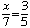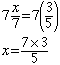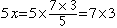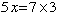Name: J E Swinton Who is asking: Student Level: Elementary Question: Why does cross multiplication work? How come canceling work? Hi, An equation is like a balance in the sense that you can add the same amount to both sides and maintain the balance. Likewise you can subtract the same amount from both sides, multiply both sides by the same amount or divide both sides by the same amount. In each case the balance is maintained. (The only problem that might arise is if you attempt to divide by zero.) Thus if you haveMultiply both sides by 7 to getNow multiply both sides by 5Hencewhich is what you would get from the first equation by cross multiplication. Cancellation works in a similar fashion. If 3*x = 3*7 (where * is multiplication) then dividing both sides by 3 gives x = 7. I hope this helps,Penny Go to Math Central